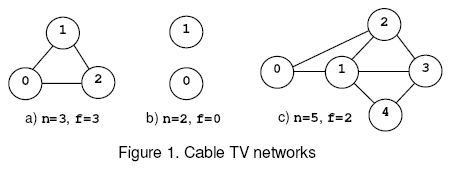# 题目描述# 代码

#include
#include
#include
//#include
#define INT_MAX 2147483645
#include
#include
#include
#include
#include
#include
#include
#include
#include

#define Re register
#define LL long long
#define U unsigned
#define FOR(i,a,b) for(Re int i = a;i <= b;++i)
#define ROF(i,a,b) for(Re int i = a;i >= b;--i)
#define SFOR(i,a,b,c) for(Re int i = a;i <= b;i+=c)
#define SROF(i,a,b,c) for(Re int i = a;i >= b;i-=c)
#define CLR(i,a) memset(i,a,sizeof(i))
#define BR printf("--------------------\n")
#define DEBUG(x) std::cerr << #x << '=' << x << std::endl
#define int LL
const int MAXN = 1000+5;
const int MAXM = 20000+5;

struct Node{
struct Edge *first,*cur;
int level,num;
}node[MAXN];

struct Edge{
Node *s,*t;
Edge *next,*rev;
int cap,flow;
}pool[MAXM<<1],*frog = pool;

Edge *New(Node *s,Node *t,int cap){
Edge *ret = ++frog;
*ret = (Edge){s,t,s->first,NULL,cap,0};
return ret;
}

inline void add(int u,int v,int cap){
// printf("%d %d %d\n",u,v,cap);
node[u].first = New(&node[u],&node[v],cap);
node[v].first = New(&node[v],&node[u],0);
node[u].first->rev = node[v].first;
node[v].first->rev = node[u].first;
}

int S,T,N;

bool bfs(Node *s,Node *t){
FOR(i,0,N){
node[i].cur = node[i].first;
node[i].level = 0;node[i].num = i;
}
std::queue q;q.push(s);
s->level = 1;
while(!q.empty()){
Node *v = q.front();q.pop();
for(Edge *e = v->first;e;e = e->next){
if(e->flow < e->cap && !e->t->level){
e->t->level = v->level + 1;
if(e->t == t) return true;
q.push(e->t);
}
}
}
return false;
}

int dfs(Node *v,Node *t,int limit=INT_MAX){
if(v == t) return limit;
int flow;
for(Edge *&e = v->cur;e;e = e->next){
if(e->flow < e->cap && e->t->level == v->level + 1){
if((flow = dfs(e->t,t,std::min(limit,e->cap-e->flow)))){
e->flow += flow;
e->rev->flow -= flow;
return flow;
}
}
}
return 0;
}

int dinic(){
int flow,ans=0;
for(Edge *e = pool;e != frog+1;e++) e->flow = 0;
while(bfs(&node[S],&node[T])){
while((flow = dfs(&node[S],&node[T]))){
ans += flow;
}
}
return ans;
}

inline void init(){
CLR(node,0);frog = pool;
}

int n,m;

inline void Solve(){
init();
FOR(i,1,m){
int u,v;
scanf(" (%lld,%lld)",&u,&v);//u++;v++;
}
S = n;N = (n<<1);
int ans = n;
FOR(i,1,n-1){
T = i;//DEBUG(ans);
ans = std::min(ans,dinic());
}
printf("%lld\n",ans);
}
/*
1 2
1 3
2 3
*/

signed main(){
while(~scanf("%lld%lld",&n,&m)) Solve();
return 0;
}
/*
1 4 1
2 5 1
3 6 1
4 2 100000000
5 1 100000000
4 3 100000000
6 1 100000000
5 3 100000000
6 2 100000000
*/


Last modification：April 7th, 2020 at 10:14 pm